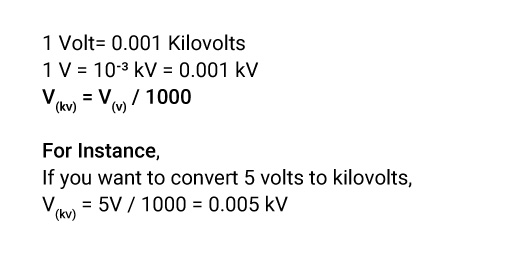## About Our V to kV Converter

“Do many calculations to lead to victory and few calculations to defeat.”

Metric conversion has always been confusing in terms of units and numbers. Confusion leads to erroneous calculations. So, we are presenting a quick metric converter for you, which will give you a quick and precise outcome regardless of the unit and size of the number.

Whether you want to switch volts to kilovolts or looking for other metric conversions, use this tool to ease your query and save time.

### How to Convert Volts to Kilovolts?

If you want to convert volts to kilovolts, you will have to divide the potential difference (Voltage) by the conversion ratio. A voltage in kilovolts is equal to the volts divided by 1000.

One volt is equal to 0.001 kilovolts.

Voltage is the potential difference created when one ampere of current is passed through one ohm of resistance.

### The Volts to Kilovolts Conversion Formula.

Here is the volt-to-kilovolts conversion formula, which you can use for direct calculation.If you don’t want to get stuck in the formulas and lengthy calculations, you can use our handy volts-to-kilovolts calculator to get the answer in a fraction of a second.

### How to Use Our Volts to Kilovolts Converter?

Our volts-to-kilovolts converter is pretty easy to use. Grab the answer within a second.

• Step1: Enter our website.
• Step2: Put the voltage value to be converted into kilovolts.
• Step3: Click on “Convert” to get the answer.

### How Many Volts Are in Kilovolts?

There are 1000 volts in one kilovolt.

1 Kilovolt = 1000 Volts

1Kv= 103 V

### What is the Difference Between Volts & Kilovolts?

Volts and Kilovolts, both units, are used to measure the potential difference (Voltage). But here is the difference between volts and kilovolts.

Volts Kilovolts
Volts is represented by V Kv represents Kilovolts
It is usually used in electrical engineering. Kilovolts are primarily used in scientific areas.
Measures the low-voltage circuits Measure the high-transmission lines.
1 volt= 0.001 Kv 1 Kv= 1000 Volts

### Examples of Converting Volts to Kilovolts.

Just flip through the examples to get a clear idea to convert Volts to Kv.

V(kV) = 3V / 1000 = 0.003 kV

V(kV) = 6V / 1000 = 0.006 kV

V(kV) = 7V / 1000 = 0.007 kV

V(kV) = 10V / 1000 = 0.01 kV

### Volt to Kilovolts Conversion Table.

Here is an ideal volt-to-kilovolts conversion table to understand the pattern.

Volts Kilovolts
1V 0.001Kv
10V 0.01Kv
100V 0.1Kv
1000V 1Kv